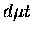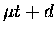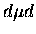Next: Sticking and stripping Up: Overview of the muon Previous: Resonant formation

## Nuclear fusion

The rate of nuclear fusion in themolecule is of the order of 1012 s-1, hence it is too fast to measure experimentally. The reaction is strongly dominated by the resonance 5He, which lies about 55 keV abovethreshold. Because of the centrifugal barrier of the J=1 states of the molecule, fusion is expected to take place mainly from J=0 states, i.e., (J,v)=(0,1) and (0,0), into which the initial state (1,1) is quickly converted via fast E1Auger transitions.

For, on the other hand, the transition fromis strongly suppressed (since it requires spin flip, analogous to the ortho-para transitions in the homonuclear hydrogen molecule), and fusion takes place mainly from the J=1 states, if the molecule is resonantly formed in the (1,1) state. This in fact offers a unique opportunity to study the p wave fusion reaction at very low energy, which is difficult to do in a beam experiment. An interesting feature of this reaction is that the fusion width ratio of the (n+ 3He) channel to the (p + t) channel is about 1.4, apparently violating charge symmetry.

In a simple approach, the fusion rate is calculated by treating three-body Coulomb physics (molecular wave functions) and nuclear physics separately , a method known as factorization. For, we have(10)

where A is related to the zero energy limit of the astrophysical S factor,(11)

withbeing the reduced mass of d and t. S is obtained from the fusion cross section with Coulomb repulsion factored out, hence it contains all the nuclear physics. Thus in this approach, the overlap of the d-t wave functions in the united-atom limit is multiplied by a single number describing the strong interaction.

More accurate approaches treat the nuclear force dynamically by directly incorporating it as a complex potential in the three-body Hamiltonian (optical potential method) [55,56], or as complex boundary conditions at the nuclear surface (R-matrix method) [57,58,59]. These elaborate approaches agree rather well with each other, as well as with the simple factorization approach.

Incollisions, fusion in flight'', i.e., without forming a boundmolecule, is also possible, and its rates have been calculated by various methods including, most recently, Faddeev equations (see  and references therein). The reactions are enhanced, compared to bare nuclear collisions, especially if virtual states exist, due to the increased overlap of the nuclear wave functions.Next: Sticking and stripping Up: Overview of the muon Previous: Resonant formation